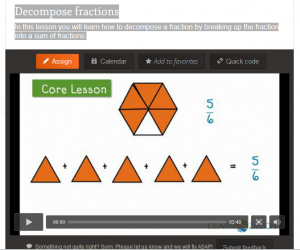Crush Classroom Boredom by running an escape room at school. Just download, print & play! Show me...

# Decomposing Fractions Video LessonsDecompose Fractions Lesson 1 – Video lesson where you will learn how to decompose a fraction by breaking up the fraction into a sum of fractions.

Decompose Fractions Lesson 2 –  Video lesson where you learn how to decompose fractions by using addition.

CCSS.Math.Content.4.NF.B.3 Understand a fraction a/b with a > 1 as a sum of fractions 1/b.
CCSS.Math.Content.4.NF.B.3a Understand addition and subtraction of fractions as joining and separating parts referring to the same whole.
CCSS.Math.Content.4.NF.B.3b Decompose a fraction into a sum of fractions with the same denominator in more than one way, recording each decomposition by an equation. Justify decompositions, e.g., by using a visual fraction model. Examples: 3/8 = 1/8 + 1/8 + 1/8 ; 3/8 = 1/8 + 2/8 ; 2 1/8 = 1 + 1 + 1/8 = 8/8 + 8/8 + 1/8.
CCSS.Math.Content.4.NF.B.3c Add and subtract mixed numbers with like denominators, e.g., by replacing each mixed number with an equivalent fraction, and/or by using properties of operations and the relationship between addition and subtraction.